Start typing, then use the up and down arrows to select an option from the list.# Energy Diagrams Concept 2

Jules Bruno
264views
4
the speed of a chemical reaction is based on the height of the activation energy. Now here, activation energy, which is your energy barrier and abbreviated as e sub A equals your transition state. So abbreviate as t s minus, you'll react in line. So we're going to say here If we're to look at these diagrams, remember, this is your transition state. This is your reactant. This is your transition state. This is your reactant. From this, we can calculate the energy of activation for both of these curves. So if we were to do that, we'd say that the transition state up here looks like it's around 90. So this would be 90, And then the reaction line looks like it touches 20. So this would be 70 killer jewels for the activation energy. Remember, it's the difference between the two And then in our other curve. The transition state looks like it's 60, And the reaction line still looks like it's around 20. So that's 40 killer jewels. Yeah, so remember, it's the difference between the two, which represents our activation energy. Now, what's important here? We're going to say that the higher the activation energy than fewer reactive molecules have enough energy to convert into products. So we're going to stay here. A higher activation energy equates to a slower reaction, and a smaller activation energy equates to a faster reaction. Here. We just calculated the activation energy for two energy diagrams, this one being 70 kill a jewels and this one being 40. So remember the one that has the higher activation energy, where represent our slower reaction, and the one that has the smaller or lower activation energy would represent art. Faster reaction. So just remember we can use activation energy to give us a good idea of how quickly or how slowly any typical chemical reaction will go. So keep this in mind when calculating activation energy.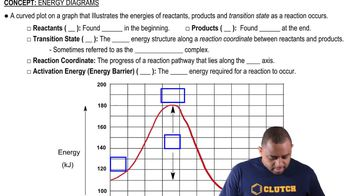01:57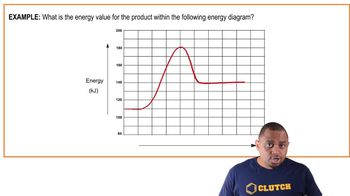01:02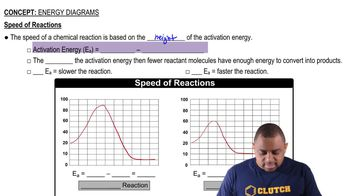02:02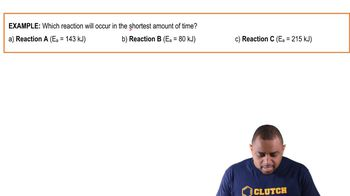00:40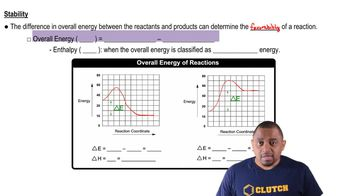03:22